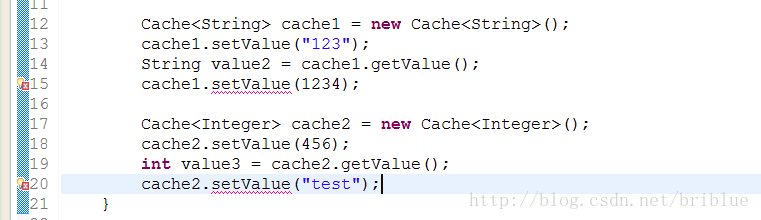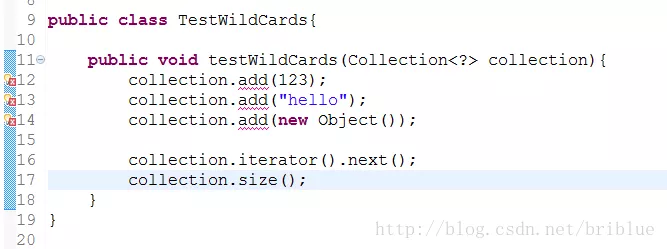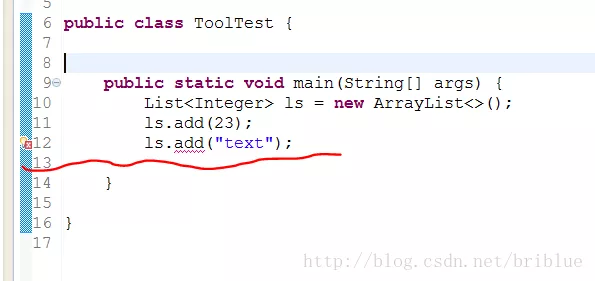<!-- more -->

List<String> l1 = new ArrayList<String>();
List<Integer> l2 = new ArrayList<Integer>();

System.out.println(l1.getClass() == l2.getClass());


# 泛型是什么？

public class Cache {
Object value;

public Object getValue() {
return value;
}

public void setValue(Object value) {
this.value = value;
}

}


Cache cache = new Cache();
cache.setValue(134);
int value = (int) cache.getValue();
cache.setValue("hello");
String value1 = (String) cache.getValue();


public class Cache<T> {
T value;

public Object getValue() {
return value;
}

public void setValue(T value) {
this.value = value;
}

}


Cache<String> cache1 = new Cache<String>();
cache1.setValue("123");
String value2 = cache1.getValue();

Cache<Integer> cache2 = new Cache<Integer>();
cache2.setValue(456);
int value3 = cache2.getValue();1. 与普通的 Object 代替一切类型这样简单粗暴而言，泛型使得数据的类别可以像参数一样由外部传递进来。它提供了一种扩展能力。它更符合面向抽象开发的软件编程宗旨。

2. 当具体的类型确定后，泛型又提供了一种类型检测的机制，只有相匹配的数据才能正常的赋值，否则编译器就不通过。所以说，它是一种类型安全检测机制，一定程度上提高了软件的安全性防止出现低级的失误。

3. 泛型提高了程序代码的可读性，不必要等到运行的时候才去强制转换，在定义或者实例化阶段，因为 Cache<String> 这个类型显化的效果，程序员能够一目了然猜测出代码要操作的数据类型。

# 泛型的定义和使用

1. 泛型类。
2. 泛型方法。
3. 泛型接口。

public class Test<T> {
T field1;
}


public class Test<Hello> {
Hello field1;
}


1. T 代表一般的任何类。
2. E 代表 Element 的意思，或者 Exception 异常的意思。
3. K 代表 Key 的意思。
4. V 代表 Value 的意思，通常与 K 一起配合使用。
5. S 代表 Subtype 的意思，文章后面部分会讲解示意。

Test<String> test1 = new Test<>();
Test<Integer> test2 = new Test<>();


public class Test<String> {
String field1;
}


public class MultiType <E,T>{
E value1;
T value2;

public E getValue1(){
return value1;
}

public T getValue2(){
return value2;
}
}


public class Test1 {

public <T> void testMethod(T t){

}
}


public  <T> T testMethod1(T t){
return null;
}


### 泛型类与泛型方法的共存现象

public class Test1<T>{

public  void testMethod(T t){
System.out.println(t.getClass().getName());
}
public  <T> T testMethod1(T t){
return t;
}
}


Test1<String> t = new Test1();
t.testMethod("generic");
Integer i = t.testMethod1(new Integer(1));


public class Test1<T>{

public  void testMethod(T t){
System.out.println(t.getClass().getName());
}
public  <E> E testMethod1(E e){
return e;
}
}


public interface Iterable<T> {
}


# 通配符 ？

class Base{}

class Sub extends Base{}

Sub sub = new Sub();
Base base = sub;


List<Sub> lsub = new ArrayList<>();
List<Base> lbase = lsub;


1. <?> 被称作无限定的通配符。

2. <? extends T> 被称作有上限的通配符。

3. <? super T> 被称作有下限的通配符。

public void testWildCards(Collection<?> collection){
}List<?> wildlist = new ArrayList<String>();


<? extends T>

<?> 代表着类型未知，但是我们的确需要对于类型的描述再精确一点，我们希望在一个范围内确定类别，比如类型 A 及 类型 A 的子类都可以。

public void testSub(Collection<? extends Base> para){

}


para.add(new Sub());


<? super T>

public void testSuper(Collection<? super Sub> para){
}


<? super T> 神奇的地方在于，它拥有一定程度的写操作的能力。

public void testSuper(Collection<? super Sub> para){
}


# 通配符与类型参数的区别

public void testWildCards(Collection<?> collection){}


public <T> void test(Collection<T> collection){}


public <T> void test(Collection<T> collection){
}


public class Test2 <T,E extends T>{
T value1;
E value2;
}

public <D,S extends D> void test(D d,S s){

}


E 类型是 T 类型的子类，显然这种情况类型参数更适合。

public <T> void test(T t,Collection<? extends T> collection){

}


public T test1(T t){
return value1;
}


# 类型擦除

List<String> l1 = new ArrayList<String>();
List<Integer> l2 = new ArrayList<Integer>();

System.out.println(l1.getClass() == l2.getClass());


public class Erasure <T>{
T object;

public Erasure(T object) {
this.object = object;
}

}


Erasure 是一个泛型类，我们查看它在运行时的状态信息可以通过反射。

Erasure<String> erasure = new Erasure<String>("hello");
Class eclz = erasure.getClass();
System.out.println("erasure class is:"+eclz.getName());


erasure class is:com.frank.test.Erasure


Class 的类型仍然是 Erasure 并不是 Erasure<T> 这种形式，那我们再看看泛型类中 T 的类型在 jvm 中是什么具体类型。

Field[] fs = eclz.getDeclaredFields();
for ( Field f:fs) {
System.out.println("Field name "+f.getName()+" type:"+f.getType().getName());
}


Field name object type:java.lang.Object


public class Erasure <T extends String>{
//  public class Erasure <T>{
T object;

public Erasure(T object) {
this.object = object;
}

}


Field name object type:java.lang.String


public class Erasure <T>{
T object;

public Erasure(T object) {
this.object = object;
}

}

}


Erasure<String> erasure = new Erasure<String>("hello");
Class eclz = erasure.getClass();
System.out.println("erasure class is:"+eclz.getName());

Method[] methods = eclz.getDeclaredMethods();
for ( Method m:methods ){
System.out.println(" method:"+m.toString());
}


method:public void com.frank.test.Erasure.add(java.lang.Object)


# 类型擦除带来的局限性public interface List<E> extends Collection<E>{

}


boolean add(Object obj);


public class ToolTest {

public static void main(String[] args) {
List<Integer> ls = new ArrayList<>();
try {

method.invoke(ls,"test");
method.invoke(ls,42.9f);
} catch (NoSuchMethodException e) {
// TODO Auto-generated catch block
e.printStackTrace();
} catch (SecurityException e) {
// TODO Auto-generated catch block
e.printStackTrace();
} catch (IllegalAccessException e) {
// TODO Auto-generated catch block
e.printStackTrace();
} catch (IllegalArgumentException e) {
// TODO Auto-generated catch block
e.printStackTrace();
} catch (InvocationTargetException e) {
// TODO Auto-generated catch block
e.printStackTrace();
}

for ( Object o: ls){
System.out.println(o);
}

}

}


23
test
42.9


# 泛型中值得注意的地方

List<int> li = new ArrayList<>();
List<boolean> li = new ArrayList<>();


List<Integer> li = new ArrayList<>();
List<Boolean> li1 = new ArrayList<>();


public <T> T test(T t){
return null;
}


test("123");


public String test(String t);


Java 不能创建具体类型的泛型数组

List<Integer>[] li2 = new ArrayList<Integer>[];
List<Boolean> li3 = new ArrayList<Boolean>[];


List<Integer> 和 List<Boolean> 在 jvm 中等同于List<Object> ，所有的类型信息都被擦除，程序也无法分辨一个数组中的元素类型具体是 List<Integer>类型还是 List<Boolean> 类型。

List<?>[] li3 = new ArrayList<?>;
li3 = new ArrayList<String>();
List<?> v = li3;